# Intro to Programming: Conditional Statements - If, Elif, and Else

In this article in my Intro to Programming series, we’re going to talk about conditional statements: if, elif and else.
By Ciprian Stratulat • Updated on Jun 21, 2022

Welcome back to the latest article in my Intro to Programming series. Today, we’re going to talk about conditional statements: if, elif and else.

## If, Elif, Else

In this article, we're going to talk about control flow. In particular, we will discuss the first control flow statement: the conditional, or if statement.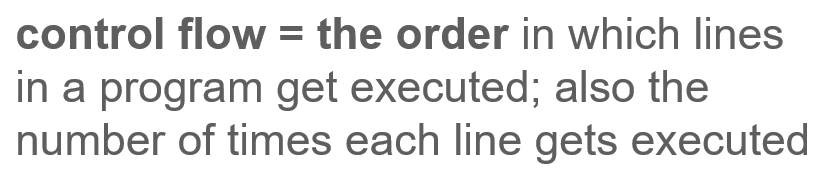By way of definition, control flow refers to the order in which lines in a computer program get executed. It's also the number of times each line gets executed.

To understand this better, let's consider the following program.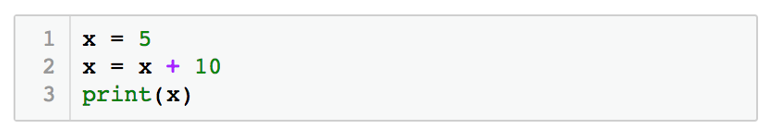Here, we define a variable x, and assign it the integer value 5. On the next line, we take the value stored in x (which is 5), add 10 to it, and then assign the result (which is 15) back to x. So after the second line, the value stored in x is 15. Finally, on the third line of code we print x. If we run this program, the output would be the integer 15.

As you see, when the Python interpreter executes this program, it reads it top to bottom and it goes line by line, executing each line exactly once. This is the default flow of any program - start at the top of the file, and read each line going all the way until the end.

However, programs would not be very powerful if this was the only “flow” they allowed. To solve many problems, we often have to execute some lines of code if and only if one or multiple conditions are met. In addition, sometimes we need to execute some lines of code multiple times.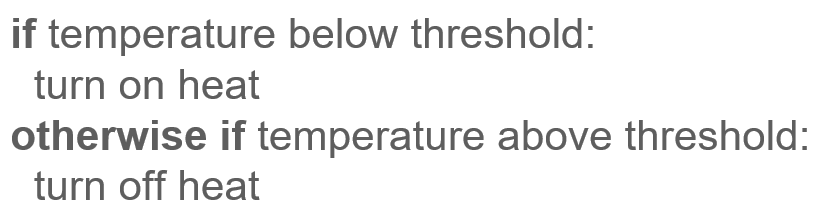For example, imagine a program that controls your home thermostat. It needs to turn on the heat if the current temperature goes below a preset value. Otherwise, if the current temperature is above a preset value, it needs to turn off the heat. Such a program needs to be able to only execute certain commands (turn on heat, turn off heat) when certain conditions are met.

In this article, I'll show you how to implement this condition-driven logical flow. To do so, we're going to learn about the Python keywords that allow us to build it.These keywords are if, elif and else. Elif here is probably the one that's odd - but think of elif as an abbreviation for "else if". In other words, elif is like “otherwise if”.

### If-Statements

So let's see what this condition-driven flow looks like. Going back to our thermostat example, let's write the part of the logic that would turn on the heat if the temperature drops below 71. We'll assume that 71 is the temperature we want to keep constant in the house.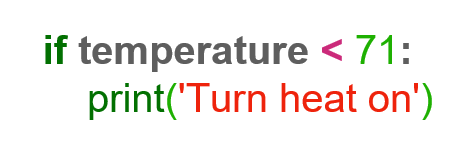As you can see here, we first start by writing the keyword if, followed by the condition that we want met. In this case, the condition is that the temperature drops below 71, or in other words, the temperature is less than 71. We then end the line with a colon, and this colon is important because it essentially tells Python that this is where the condition ends. What follows on the next line is the command that needs to be run when the condition is True. In our case, we'll use the print function to just print the message "Turn heat on".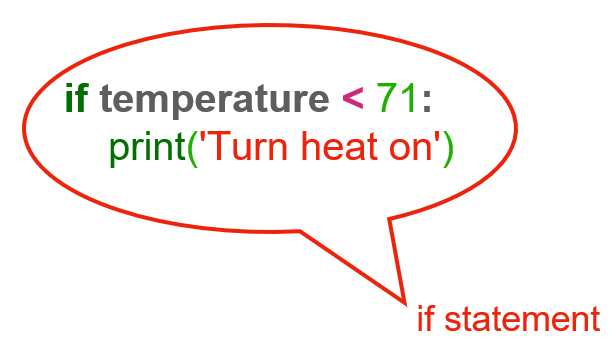This whole construct is called an "if-statement". An if-statement is one of the control flow statements available in Python, and it's the one that makes it possible to execute some lines of code if and only if some conditions are met.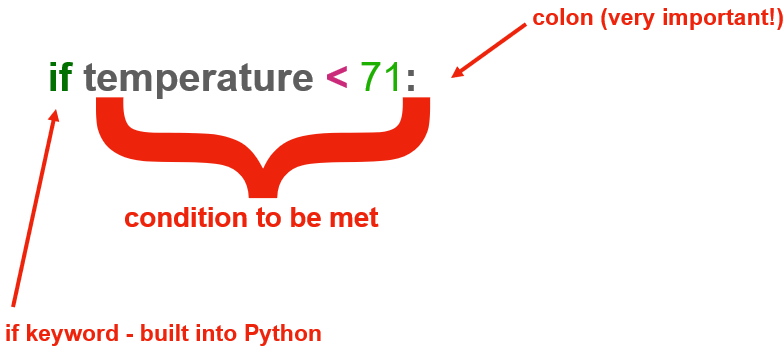Let's break this if-statement down a bit and go over the important parts. On the first line, we start with the keyword if, which is part of the Python programming language. Then, we follow with temperature < 71. This is our condition. And finally, we end with the very important colon.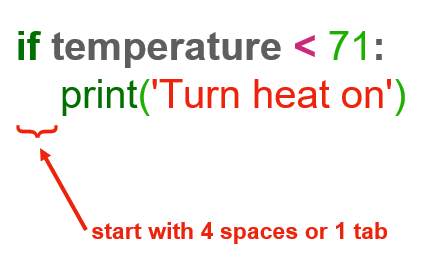On the next line, we write the command that needs to be executed when the if condition is met. In our case, it's print('Turn heat on'). You can actually have multiple lines of code here - you can even have other nested if-statements. We'll see more examples of this in just a bit.

### Indent Lines After the Condition

The important thing to remember about this line, and all other lines that depend on the same condition, is that they are indented 4 spaces, or exactly one tab.

This indentation is very important because it tells the Python interpreter that this line's "inside" the if-statement, not outside of it. That is, because of this indentation, Python knows that, if the condition above is true, then this line should be executed.

Python is very picky about white spaces, so you need to be very careful. You can only use 4 spaces or a tab. You can't use 2 spaces or 3 spaces because the interpreter will complain. Because white space is not always very visible in a text editor, it can be a bit confusing at first, because you might put 3 spaces instead of a tab, for example. The code might look good to you, but you'll get an error, and be left scratching your head. When that happens, always check your spaces. On the plus side, because Python doesn't use other special characters in building these if-statements, it's much more readable when compared to other programming languages.

### Elif Branches: Else If

Now we’ve built the first part of the logic required by our thermostat. Namely, if the temperature drops below our preset value of 71 degrees Fahrenheit, we'll turn the heat on.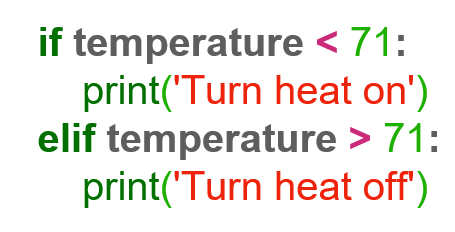Now, we need to add the second part: if the temperature goes above 71 degrees Fahrenheit, we need to turn the heat off. We can do that by adding an elif branch, as you can see here. We call these branches, because at this point, the execution of the program looks a bit like the branches of a tree or a fork in the road - you reach a point where you can go one way or another, depending on which condition is true.

This second branch of our if-statement is very similar to the first one, with a couple of exceptions. First, we start with the elif keyword, which, remember, is just short for “else if”. The condition is different too, because we want to turn off the heat if the temperature goes above 71. Notice that we still have the colon at the end. Equally important, notice that print('Turn heat off') is also indented 4 spaces or a tab. This, again, tells the Python interpreter that this line of code is inside the elif branch, so it should only be executed if the elif condition is true.

You can have as many elif branches as you need, or none at all. Inside the elif branch, you can also have a nested if-statement. We'll look at some examples of those in one of the following articles.

### Else Branches

So now, you might ask: well, what happens if the temperature is neither greater than, nor less than 71? In other words, given this if-statement, what happens if the temperature is exactly 71? Well, given this if-statement, nothing happens. Neither of the commands executes because neither of the branches have a condition that is True in that case.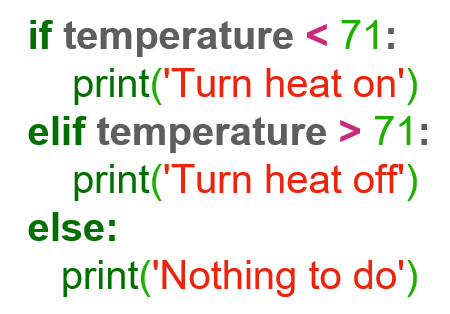If you explicitly want to handle the case of the temperature being exactly 71, you can use an else branch, like this.

The else branch always comes at the end, and doesn't check any conditions. Instead, whatever's nested inside the else branch gets executed if none of the previous branches had conditions that were true. It's basically a catch-all branch. Similar to if and elif, notice the use of a colon, and the 4 spaces or tab indentation. Again, these are essential.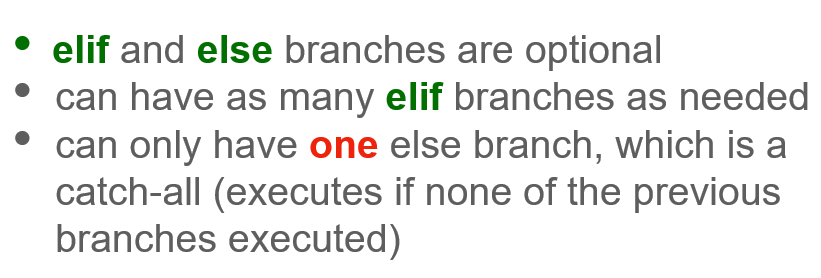Finally, I want to reiterate a few important points about the if-statement. Number one: elif and else branches are entirely optional. You can just have a standalone if branch with no elif or else branch if that's what you need. Number two: you can have as many elif branches as you need to. Maybe you have multiple situations that you need to test for. We'll see some examples of that in a second. And finally, the else branch can only show up at most once in an if-statement, and it must be the last branch. It's basically a catch-all kind of branch, and the code lines nested inside it execute if and only if none of the previous branches had conditions that were met.

Let’s do a hands-on exploration of the conditional statement in Python. Let's take a look at some code.

### How to Write an If-Statement

Let's start by writing the thermostat example. We'll define a variable that stores the current temperature, and let's set that to 60. Now, we can write the first condition: if the temperature is < 71, print turn heat on. Notice how after the colon, when I hit enter, the Jupyter notebook automatically added that indentation that I mentioned earlier. That's just Jupyter being helpful. If you're using other programming editors, chances are they too will help you with the indentation.

``````# Let's define our variable
temperature = 60

# And now, let's create our if-statement
if temperature < 71:
print('Turn heat on')
# Our input will be Turn heat on``````

If I run this code, I'll see the output 'turn heat on', which is correct, because the current temperature is too low (60 is less than 71). Now, if I go back and change the value stored in the temperature variable to 75 and run the code again, I see that nothing gets printed. That makes sense. Basically, the condition in the if-statement was not met, because 75 is not less than 71, and so the line below, where we print, never got executed.

``````# Let's adjust our variable
temperature = 75

# If we run our code again
if temperature < 71:
print('Turn heat on')
# Nothing is printed``````

### How to Write an Elif Branch

Let's add the elif branch: elif temperature > 71, print(‘turn heat off’). If we run this, we get the output turn heat off, which also makes sense. The temperature is 75, which is greater than 71, so we need to turn the heat off.

``````# Let's add our elif branch to our code
temperature = 75

if temperature < 71:
print('Turn heat on')
elif temperature > 71:
print('Turn heat off')
# Our output will be Turn heat off``````

### How to Write an Else Branch

Finally, let's add the else branch as well: else print('nothing to do'). If I run it again, the output is still Turn heat off and that's because the current temperature is still 75. Let's make that 71 and run the code again. Now we get Nothing to do.

``````# Let's add our else statement
temperature = 75

if temperature < 71:
print('Turn heat on')
elif temperature > 71:
print('Turn heat off')
else:
print('Nothing to do')
# Our output is still Turn heat off

#But if we adjust our variable to 71
temperature = 71

if temperature < 71:
print('Turn heat on')
elif temperature > 71:
print('Turn heat off')
else:
print('Nothing to do')
# Now our output is Nothing to do``````

Notice again how if, elif and else are aligned - there's no indentation here. What happens if, for example, I accidentally add one space before this elif branch? Let's try it. As you can see, I get an IndentationError: unindent does not match any outer indentation level. So pay attention to indentation. Let's fix this and run it again.

``````# If we add a space before the elif statement
if temperature < 71:
print('Turn heat on')
elif temperature > 71:
print('Turn heat off')
else:
print('Nothing to do')
# We get the following error:
# IndentationError: unindent does not match any outer indentation level``````

### How to Use If, Elif, and Else Together

Now, let's look at another example. Let's say we have a variable that stores the current day and let's set that to Monday. I'm now going to say, if the current day is a Monday, I will print meh. Else, I'll print yay. When I run this, the output will be meh because the current day, in this example, is a Monday.

``````# First, let's set our variable
current_day = 'Monday'

# Now let's write our code
if current_day == 'Monday':
print('meh')
else:
print('yay')
# Our output will be meh``````

Let's make that a bit more nuanced. Let's say that, if it's a Wednesday, we print hump day, and if it's a Thursday or a Friday we print almost weekend. To do that, we need two elif branches. On the first branch, we write elif current_day == 'Wednesday', print 'hump day'. On the second elif branch, the condition is met if the day is either Thursday or Friday, so we write elif current_day == 'Thursday' or current_day == 'Friday' print('almost weekend'). Notice here the OR logical operator, which we learned about in a previous article.

``````# Let's define our variable again
current_day = 'Monday'

#and write our code again
if current_day == 'Monday':
print('meh')
elif current_day == 'Wednesday':
print('hump day')
elif current_day == 'Thursday' or current_day == 'Friday':
print('almost weekend')
else:
print('yay')
# Our output will still be meh ``````

If we run this again, we get meh, because the day is still set to Monday. If we change the current day to Wednesday and run it again, we get hump day, as we'd expect. Finally, let's change it to Friday. When we run it again, we get almost weekend. And, of course, if we change the current day to Tuesday, we get yay because none of the previous conditions are met, so the else branch executes instead.

``````# Let's set our variable to Wednesday
current_day = 'Wednesday'

if current_day == 'Monday':
print('meh')
elif current_day == 'Wednesday':
print('hump day')
elif current_day == 'Thursday' or current_day == 'Friday':
print('almost weekend')
else:
print('yay')
# Now our output is hump day

# And if we change our variable to Friday
current_day = 'Friday'

if current_day == 'Monday':
print('meh')
elif current_day == 'Wednesday':
print('hump day')
elif current_day == 'Thursday' or current_day == 'Friday':
print('almost weekend')
else:
print('yay')
# Now our output is almost weekend

# And if we change it to Tuesday
current_day = 'Tuesday'

if current_day == 'Monday':
print('meh')
elif current_day == 'Wednesday':
print('hump day')
elif current_day == 'Thursday' or current_day == 'Friday':
print('almost weekend')
else:
print('yay')
# Now our output is yay``````

### Using Multiple Lines per Branch

Next, I want to show you an example that involves more than one code line per branch. Let's say we have a variable x and let's set that to the integer value 10. Next, let's say we want to do different mathematical operations depending on the value of x. So let's say if x is equal to 10, we want to multiply it by 2 and print the result. We write if x == 10: and then on the next line we multiply x by 2 and store the result in the same variable x. Then, on the following line, we print x. Let's run this real quick. As we might expect, the output is 20, because x is indeed equal to 10, so the condition is met and these lines of code inside the if-statement will execute, essentially multiplying 10 by 2 and printing the result.

``````# Let's set our variable
x = 10

# and write our if-statement
if x == 10:
x = x * 2
print(x)
# Our output will be 20``````

Let's add another branch and say elif x > 10:. If this is true, we'll subtract 2 from x and print the result, so x = x - 2 and print(x). If we run this, we again get 20 because x was still defined as 10 before the if-statement. Let's go and change x, and set it to 15. As this is greater than 10, when we rerun the code, the result will be 13. That's because this time, the condition on the elif branch is True, so we subtract 2 from 15 and we get 13.

``````x = 10

# Let's add an elif branch to our code
if x == 10:
x = x * 2
print(x)
elif x > 10:
x = x - 2
print(x)
# Our outcome is 20

# If we change our variable to 15
x = 15

if x == 10:
x = x * 2
print(x)
elif x > 10:
x = x - 2
print(x)
# Our output is now 13``````

I went through these examples to show you that you can have as many lines of code on each branch as you need. The only thing you need to do is make sure that all of them start with the correct indentation.

## Nested If-Statement

Let’s explore the concept of if-statements within if-statements. In other words, nested if-statements.

If-statements are a very flexible construct in Python. I mentioned earlier that, in each branch of the if-statement, you can nest other if-statements. Let me show you an example of that. Let's write some code that, when the weather is nice and it's the weekend, it will tell me to go out. When the weather is nice and it's not the weekend, it will tell me to go to work, and when the weather is not nice it will tell me to stay home.

First, the definition of what nice weather is can be subjective, but we can just define a boolean variable. Let's say weather_is_nice, and I'll set it to True for now. In addition, let's have a current day variable, and this will, of course, represent the current day of the week. Let's say that current_day = 'Saturday', which is indeed a weekend day.

So now, I can write: if weather_is_nice is True, and it's the weekend, so current_day is either 'Saturday' or 'Sunday', print 'Go out'. Notice how we used the parentheses here around current_day == 'Saturday' or current_day == 'Sunday'. That's because this condition tells us if it's a weekend or not. If we run this, we'll get the output go out, which makes sense because we said the weather is nice and the current day is 'Saturday'.

``````# Let's set our variables
weather_is_nice = True
current_day = 'Saturday

# Let's write our code
if weather_is_nice == True and (current_day == 'Saturday' or current_day == 'Sunday'):
print('go out')
# Our output will be go out``````

We can actually simplify this a bit. Whenever you have a variable that stores a boolean, you don't actually need to write the == in the if statement. So we can rewrite the condition as if weather_is_nice and (current_day == 'Saturday' or current_day == 'Sunday'). That's exactly the same thing. We run this again, and again the program tells us to go out because it's nice outside and it's the weekend. Sounds like a good idea!

``````weather_is_nice = True
current_day = 'Saturday'

# Let's simplify our code a bit
if weather_is_nice and (current_day == 'Saturday' or current_day == 'Sunday):
print('go out')
# Our output is still go out``````

Now, we had two other conditions: if the weather is nice and it's not the weekend, we need to go to work. So I'm going to add an elif branch here and write elif weather_is_nice and not (current_day == 'Saturday' or current_day == 'Sunday') print('go to work'). So, here we use the NOT logical operator to check if a day is not a weekend day and therefore it's a weekday. This basically says if it's not true that the current day is Saturday or Sunday, then it is true that the current day is a weekday. Let's now change current_day above, set it to 'Tuesday' and run the code again. And, as we expect, the program tells us we should go to work.

``````# Let's set our variables
weather_is_nice = True
current_day= 'Tuesday'

# Let's add our elif branch
if weather_is_nice and (current_day == 'Saturday' or current_day == 'Sunday'):
print('go out')
elif weather_is_nice and not (current_day == 'Saturday' or current_day == 'Sunday'):
print('go to work')
# Our output is go to work``````

Finally, if the weather is not nice, we are to stay home, regardless of whether it's the weekend or not. We add in this condition by writing elif not weather_is_nice:' print('stay_home'). We can go above and change weather_is_nice and set it to False. If we run this code now, we get a recommendation to stay home.

``````# Let's update our variables
weather_is_nice = False
current_day = 'Tuesday'

# And now let's update our code
if weather_is_nice and (current_day == 'Saturday' or current_day == 'Sunday'):
print('go out')
elif weather_is_nice and not (current_day == 'Saturday' or current_day == 'Sunday'):
print('go to work')
elif not weather_is_nice:
print('stay home')
# Our output will be stay home``````

So this works, but we can still improve it a bit. Notice how, in the if and first elif branches, we first check if the weather is nice. We can rewrite this program so that it works like this: if weather is nice, do something; if weather is not nice, do something else. And then, inside the weather is nice branch, use another if statement to check if it's a weekend or not, and act accordingly.
This would look like this:

``````# Let's keep our variables
weather_is_nice = False
current_day = 'Tuesday'

# Now let's simplify our code
if weather_is_nice:
if (current_day == 'Saturday' or current_day == 'Sunday'):
print('go out')
else:
print('go to work')
else:
print('stay home')
# Our output should still be stay home``````

So here, again, we set weather_is_nice to False, and the current_day to Tuesday. At the top level, we have a much simpler if - else statement. If weather_is_nice, do something. Else, do otherwise, print 'stay home'. We don't have to check again to see if the weather is not nice because, in our binary world here, the weather can either be nice or not nice. So, if the condition on the if-branch is false, it automatically implies that the weather is not nice. Now, inside the if branch, if the weather is nice, we again have two situations: if it's the weekend, we need to go out, else (so if it's not the weekend), we go to work.

Spend some time digesting this and make sure you understand it. It can look a bit daunting at first, but the nested-if statement works very much like the outside if statement. Notice that the if and else there still need to be aligned, and the code lines on each of those branches are still indented relative to the if and else keywords. If you get confused, remember that each elif or else keyword goes with the if that matches its indentation.

Let's compare these two ways of writing this program. You will hopefully see that, from a logical standpoint, the second one is a bit easier to follow, because we don't have a lot of logical operators. In addition, it also involves a bit less typing because we don't repeat some of the conditions as much. So generally, the second way is preferred.

That's all on the topic of if-statements. These control flow statements are fundamental code structures that allow us to execute parts of our program if and only if some conditions are met. They are extremely powerful and flexible, and you'll use them in any program that's sufficiently complex, so spend some time playing with them. In addition to the exercises we provided, you can design your own examples. Feel free to try out different configurations, levels of nesting, or conditions, until you're comfortable expressing in code any if-else condition you can think of.

Thank you for following along! In my next article, we’ll discuss for loops.# Ciprian Stratulat

Ciprian is a software engineer and the CTO of Edlitera. As an instructor, Ciprian is a big believer in first building an intuition about a new topic, and then mastering it through guided deliberate practice.

Before Edlitera, Ciprian worked as a Software Engineer in finance, biotech, genomics and e-book publishing. Ciprian holds a degree in Computer Science from Harvard University.